# How to create Excel formulas with ChatGPT?

Excel formulas with ChatGPT: Not everyone is proficient in using Excel spreadsheets, and it can be challenging to determine which formulas are required to manipulate your data. Fortunately, there’s a new solution that can assist you in creating the appropriate formula for your data in just a few simple steps.

This solution is ChatGPT, a cutting-edge AI chatbot developed by OpenAI. ChatGPT is capable of performing a wide range of tasks, including creating Excel formulas. In this tutorial, we’ll walk you through the process of using ChatGPT to generate the formulas you need for your Excel data. So, let’s dive in and get started.

## How to create Excel formulas with ChatGPT?

In this section, we’ll explore how to use ChatGPT to compose Excel formulas. To demonstrate this process, we’ll employ a straightforward sample data set and perform simple calculations using the formulas provided by ChatGPT. This guide aims to provide you with a clear understanding of the steps involved in generating Excel formulas with ChatGPT. So, let’s begin by examining how ChatGPT can be leveraged to simplify the formula creation process.

Before getting started with ChatGPT, there are a couple of prerequisites you’ll need to have in place. First and foremost, you’ll need an OpenAI account, which will enable you to access the chatbot’s features. Additionally, you’ll need a Microsoft Excel spreadsheet containing the data set that you’ll be working with. Without these two elements, it will not be possible to utilize the full range of ChatGPT’s capabilities for creating Excel formulas. So, make sure you’ve got your OpenAI account set up and your data set loaded into Excel before proceeding.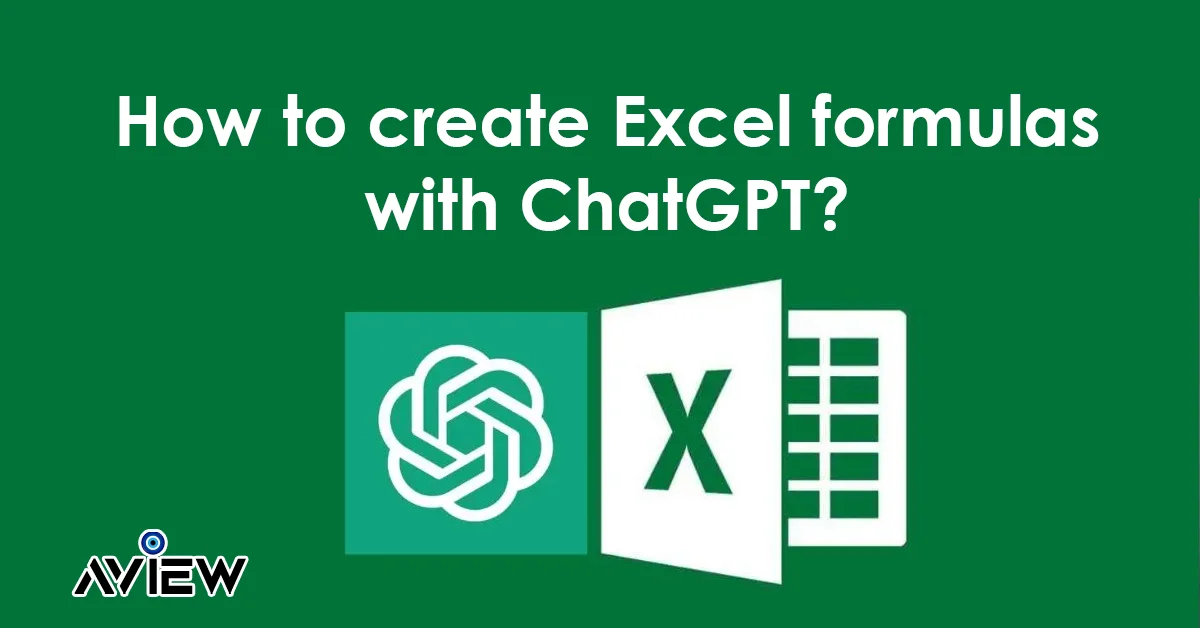Step 1: This involves opening the Excel spreadsheet that you want to work with. For our example, we have a basic table that includes products, services, projected prices, and actual prices. Our goal is to use the formulas generated by ChatGPT to calculate the variations between the projected and actual prices for each item listed in Column D. Additionally, we aim to obtain the subtotals of Columns B, C, and D. This exercise will illustrate how ChatGPT can be used to simplify the process of formula creation in Excel.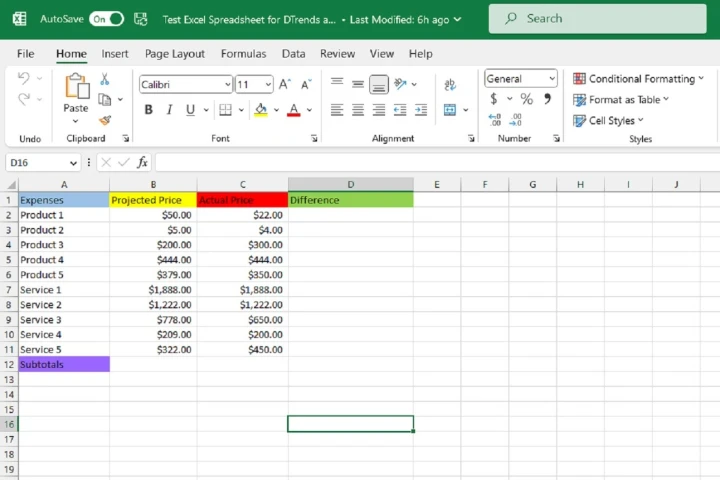Step 2: you’ll need to open your web browser and navigate to the ChatGPT website. Once you’re on the site, you’ll need to sign in to your OpenAI account to access ChatGPT’s features. After logging in, you’ll be directed to the New chat screen, where you can begin your conversation with ChatGPT.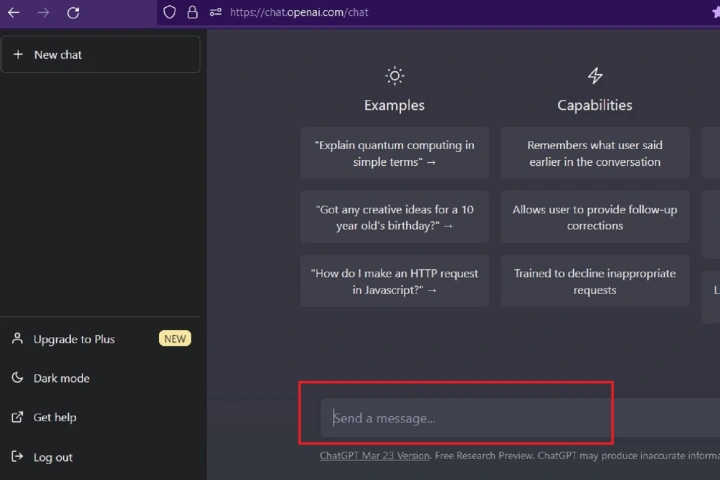Step 3: where you’ll communicate with ChatGPT to request the Excel formula you need for your data set. To do this, you’ll need to type your request into the “Send a message…” text box at the bottom of the chat screen and then press Enter on your keyboard. It’s crucial to provide a clear and concise message to ChatGPT, so it understands precisely what formula you require. Otherwise, it may provide you with the wrong formula.

In our example, we want to use the formula to calculate the differences between the values in Column B (Projected Price) and Column C (Actual Price), which we’ll input into Column D (Differences). We’ll begin by asking ChatGPT to create a formula that subtracts the value in cell C2 from the value in B2. So, our message to ChatGPT would be: “Please write an Excel formula that subtracts the value in C2 from the value in B2.”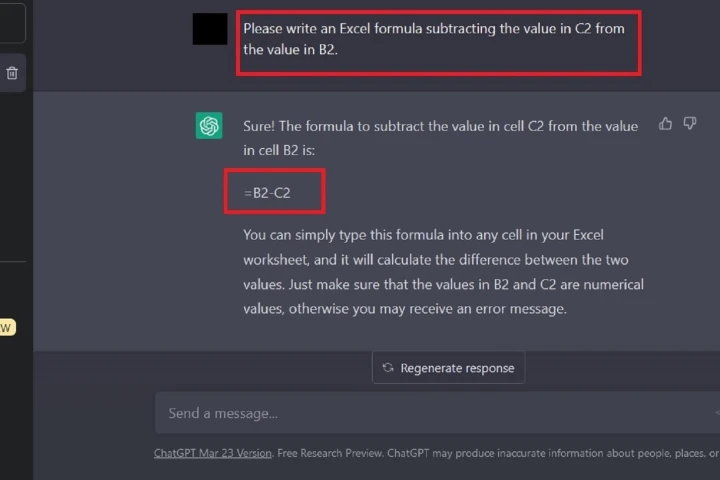Step 4: After you’ve made your request to ChatGPT for an Excel formula, you should receive a response that includes the formula you need to use, along with a brief explanation of how to implement it in your Excel spreadsheet. You can copy the formula provided by ChatGPT from the chat screen by highlighting it and then pressing CTRL + C on your keyboard.

Step 5: you’ll need to go to your Excel spreadsheet and select the cell where you want the formula to appear. Once you’ve selected the cell, you can paste the formula that you copied from ChatGPT into the cell by pressing CTRL + V on your keyboard. The correct answer to your formula should appear in the cell you selected.

In our example, the formula generated by ChatGPT was “=B2-C2”. After copying and pasting this formula into cell D2, we were able to see the correct answer for the difference between Product 1’s Projected Price (B2 which equals \$50) and its Actual Price (C2 which equals \$22), which was \$28.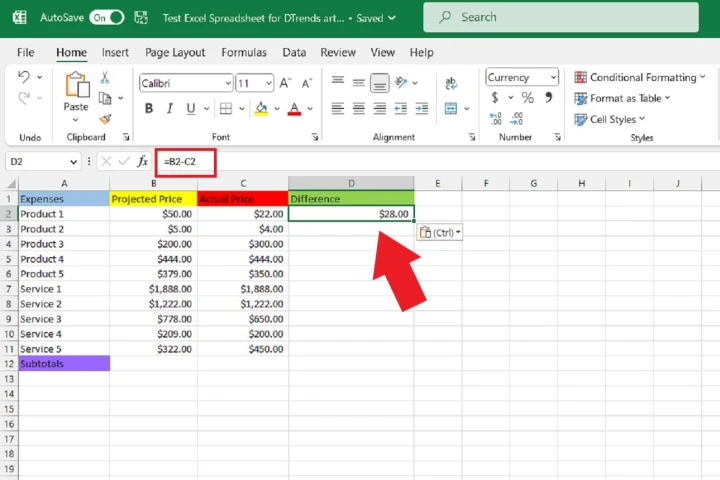Step 6: Involves copying the formula from the first cell to the remaining cells of the Differences column. You can do this by hovering the cursor over the lower right corner of the cell where you pasted the formula. You will see a black plus sign icon appear. Click and drag the cursor down to select the remaining cells in the Differences column until the last row of data. When you release the cursor, the formula will be copied to the remaining cells and the correct answers will appear in each respective cell.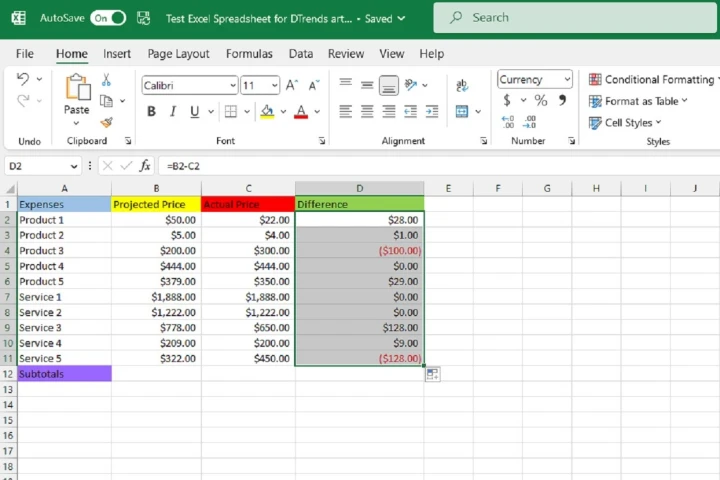Step 7: we want to use ChatGPT to generate an Excel formula that calculates the sum of all the values in Column B (Projected Price). To do this, we need to go back to the ChatGPT website and send a message with our request.

For our example, we sent the following message to ChatGPT: Please write an Excel formula that adds up all the values in Column B, from B2 to B11. It’s essential to be as specific and clear as possible in your request to ChatGPT, so the chatbot can generate the correct formula. After a few seconds, ChatGPT should respond with a formula that calculates the sum of the values in Column B.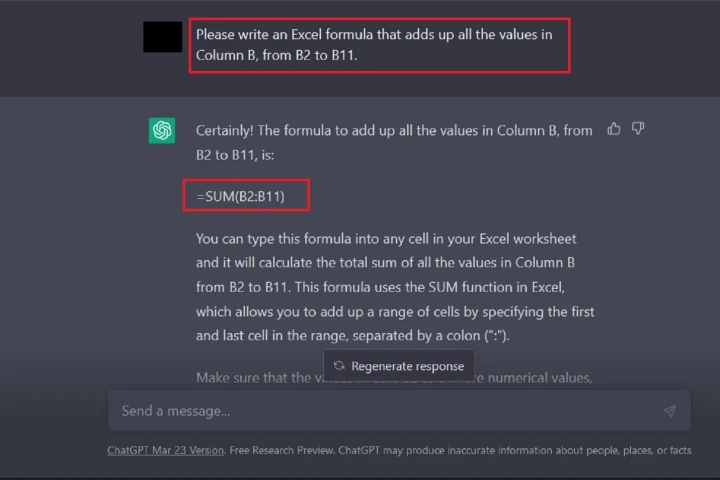Step 8: Once ChatGPT responds to your request for an Excel formula that adds up all the values in a column, you should copy and paste the formula into a desired spreadsheet cell. The formula provided by ChatGPT will then automatically calculate the correct answer and display it in the cell.

For instance, to find the sum total of all the values in Column B of the example spreadsheet, ChatGPT provided the formula =SUM(B2:B11). Once we copied and pasted this formula into a cell, the answer, \$5,497, was immediately calculated and displayed. It’s important to note that you should carefully word your request to ChatGPT, so it can accurately understand and provide the correct formula.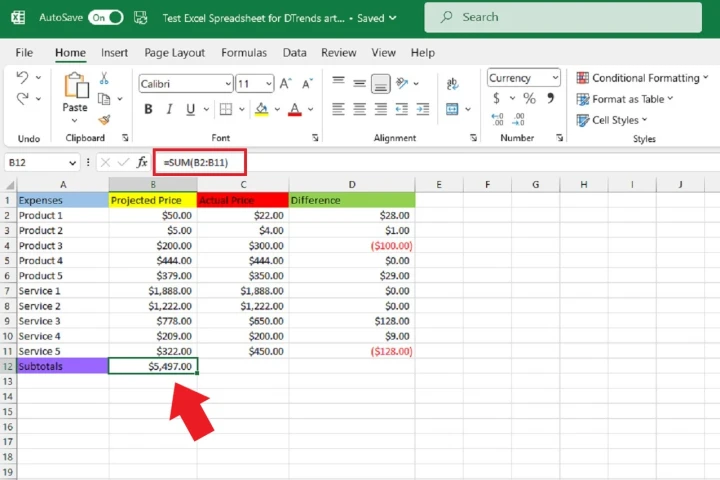Step 9: In this final step, we will fill in the subtotals of columns C and D. Similar to the previous step, we’ll hover our cursor over the lower right corner of the cell that contains the subtotal of column B until we see the black Plus sign icon appear. Then, we’ll select the corner and drag our cursor to the right to select the next two cells for columns C and D.

Upon releasing the cursor, the correct subtotal answers for columns C and D should automatically appear in their respective cells. This completes the task of calculating the differences and subtotals of a set of data using Excel formulas with the help of ChatGPT.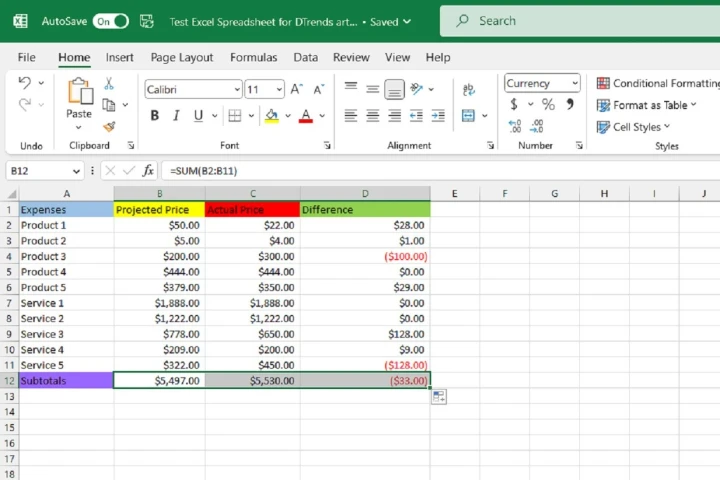## FAQs on How to create Excel formulas with ChatGPT

How do I use ChatGPT to create an Excel formula?

First, you need to log into your OpenAI account and open the ChatGPT website. Then, on the new chat screen, type in your request for an Excel formula in the “Send a message…” text box at the bottom of the screen. Be sure to word your request as clearly and precisely as possible. ChatGPT should respond with a formula and an explanation of how to use it. You can then copy and paste that formula into your desired spreadsheet cell.

What if ChatGPT doesn’t give me the right formula?

It’s important to be clear and specific in your request to minimize the chances of ChatGPT misunderstanding you. If you’re unsure, you can try rephrasing your request or providing more context. If ChatGPT still doesn’t give you the right formula, you can try asking again or seeking assistance elsewhere.

Can ChatGPT help me with other Excel tasks besides creating formulas?

Yes, ChatGPT can assist with a variety of Excel tasks, including formatting, data analysis, and more.

Is ChatGPT free to use?

OpenAI offers a limited number of free API requests per month, but there may be additional fees for higher usage.